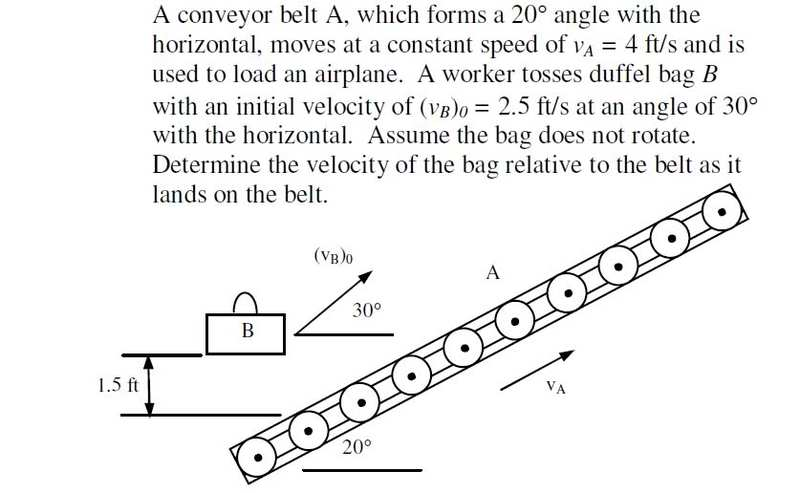# Dynamics - Relative Motion Help?

• Khamul
I was thinking maybe I should place it at the point where the duffel bag touches the conveyor belt.In summary, the problem involves determining the velocity of a duffel bag relative to a conveyor belt as it lands on the belt. The angle and speed of the conveyor belt and the initial velocity and angle of the bag are given. Using equations for x and y components, the final distance and time are calculated. To find the relative velocity, the x and y components of the final velocity are determined and then subtracted from the velocity of the belt.

## Homework Statement

Conveyor belt A, which forms a 20° angle with the horizontal, moves at a constant speed of 4 ft/s and is used to load an airplane. Knowing that a worker tosses duffel bag B with an initial velocity of 2.5 ft/s at an angle of 30° with the horizontal, determine the velocity of the bag relative to the belt as it lands on the belt.

## Homework Equations

Vi,x = Vi*cos($$\theta$$)
Vi,y = Vi*sin($$\theta$$)
(xf-xi) =Vi,x*t
(yf-yi) =Vi,y*t - (1/2)*g*t2
rB = rA+rB/A
vB = vA+vB/A
aB = aA+aB/A

## The Attempt at a Solution

I think I've gotten the majority of this problem tackled, so I will list what I have done (as I am highly likely to have done something wrong.) As you can see from the picture below, a linear equation (I think?) is required out of the conveyor belt, so that is the first thing I did.

We know that to make a right triangle with the belt, one side is 90, we're given 20, so the final angle is 70. For pure relations' sake to find a slope, I set the bottom length equal to 10, used tan(20)=opp/10, and found the rise/run to be 3.64/10 which came out to be a slope of .364.

I also set my origin at the location of the duffel bag, which was 1.5 feet above the starting location of the conveyor belt line. So, for my linear equation, I found y=.364x-1.5

Then from there, (I think this is right?) I subbed my xf and yf equations into my linear equation. I was then faced with a quadratic, which, when solved for t, I found t=.3209 s. Fast forwarding a bit more after this I found my final x and y distances.

My x final distance was .6947 ft, while my y final distance was -1.2465 ft.

And...this is where I taper off. I am unsure how to proceed next. I know my final distances, the time it took to hit the conveyor belt...but I am not sure how to relate all of this to find the relative velocity of the bag to the belt.

Could anyone spare some help? I would greatly appreciate it!4. F.B.D.Hi Khamul!Khamul said:
My x final distance was .6947 ft, while my y final distance was -1.2465 ft.

And...this is where I taper off. I am unsure how to proceed next. I know my final distances, the time it took to hit the conveyor belt...but I am not sure how to relate all of this to find the relative velocity of the bag to the belt.

now you need to find the x and y components of the final velocity

to find the relative velocity, you then just subtract the velocity components of the beltbtw, why did you do all this, instead of simply saying tan20° = 0.364 ?We know that to make a right triangle with the belt, one side is 90, we're given 20, so the final angle is 70. For pure relations' sake to find a slope, I set the bottom length equal to 10, used tan(20)=opp/10, and found the rise/run to be 3.64/10 which came out to be a slope of .364.

tiny-tim said:
Hi Khamul!now you need to find the x and y components of the final velocity

to find the relative velocity, you then just subtract the velocity components of the beltbtw, why did you do all this, instead of simply saying tan20° = 0.364 ?Goood morning tiny-tim, thanks for getting around to helping me so early!Huh. Well hey, look at that! I learned something new about slopes, thank you! Also, thank you for steering me in the right directionI was burned out between this and thermodynamics by midnight last night!

I am actually working on this problem right now and my question for you is where did you place you axis in this problem?